# 廣義量詞系列：對偶性推理進階

## 2. 新型對當方陣

### 2.1 新型對當方陣的提出∀x(S(x) ⇒ P(x))
∃x(S(x) ∧ P(x))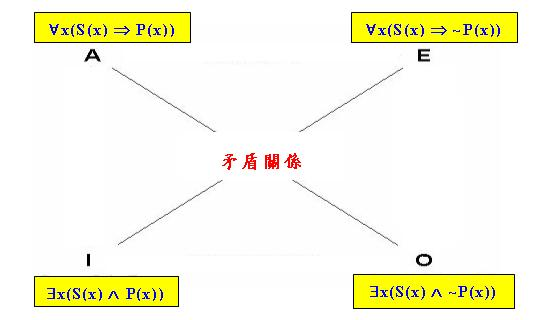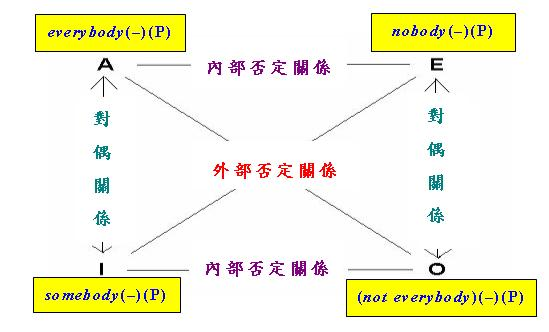everybody(−) ⇒ somebody(−) ∧ somebody(−) ~⇒ everybody(−)

everybody(−) ≡ somebody(−)dsomebody(−) ≡ everybody(−)d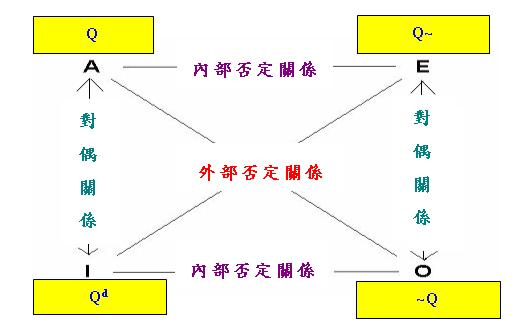「對偶性推理」並不局限於一般量詞，而是存在於很多語言結構中。舉例說，筆者在上一章便曾介紹四個「體貌副詞」的各種否定關係，現在我們可以把這四個副詞排成以下「對當方陣」：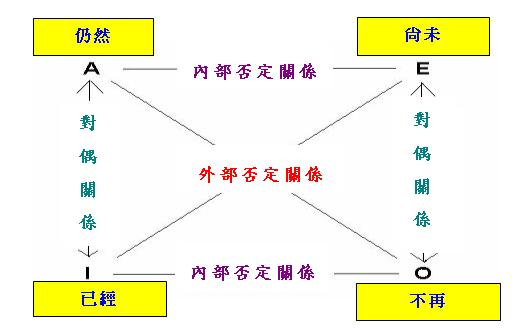### 2.2 Square函項

Square(Q) = {Q, ~Q, Q~, Qd}     (1)

Square(everybody(−)) = {everybody(−), (not everybody)(−), nobody(−), somebody(−)}

Peters和Westerstahl還證明了以下有關函項Square的定理。

 定理1： 以Square(Q)中任何一個元素作為Square的論元，所得結果等於原來的Square(Q)，即若Q' ∈ Square(Q)，則Square(Q') = Square(Q)。

 Square(~Q) = {~Q, ~(~Q), (~Q)~, (~Q)d} = {~Q, ~~Q, ~Q~, ~~Q~} = {~Q, Q, Qd, Q~} = Square(Q)

Square(everybody(−)) = Square(not everybody(−)) = Square(nobody(−)) = Square(somebody(−))

Q<1> = Square(Q1) ∪ Square(Q2) ... ∪ Square(Qn)

Square(Qi) ∩ Square(Qj) = Φ, i ≠ j

IDINONDU
IDIDINONDU
ININIDDUON
ONONDUIDIN
DUDUONINID

## 3. 自對偶

### 3.1 自對偶的概念

Q(#)(Q'(#')(Q''(#'')(B))) ⇔ Q(#)~(Q'(#')d(~Q''(#'')(B)))

QQ'Q'' ≡ Q~~Q'~~Q''

QQ'Q'' ~≡ Q~Q'~Q''

Q(#)(Q'(#')(Q''(#'')(B))) ⇔ Q(#)~(Q'(#')(~Q''(#'')(B)))     (2)

Q(#)d ≡ Q(#)     (3)

Q(#)~ ≡ ~Q(#)     (4)

### 3.2 自對偶量詞 3.2.1 專有名詞

John不穿T恤。 ⇔ 並非John穿T恤。

 (not every)({j})(B) ⇔ {j} ∩ ~B ≠ Φ ⇔ {j} ⊆ ~B ⇔ {j} ∩ B = Φ ⇔ no({j})(B)

All except one boy gave Mary at least 2 roses. ⇔ Exactly one boy gave Mary fewer than 2 roses.

### 3.2.2 反身代名詞

[self1(−)]2(B) = {(x, z): B(x, x, z)}

[~self1(−)]2(B) = ~([self1(−)]2(B)) = ~{(x, z): B(x, x, z)}
[self1(−)~]2(B) = [self1(−)]2(~B) = {(x, z): ~B(x, x, z)}

 (x, z) ∈ [~self1(−)]2(B) ⇔ (x, z) ∈ ~{(x, z): B(x, x, z)} ⇔ (x, z) ~∈ {(x, z): B(x, x, z)} ⇔ ~B(x, x, z) ⇔ (x, z) ∈ {(x, z): ~B(x, x, z)} ⇔ (x, z) ∈ [self1(−)]2(~B) ⇔ (x, z) ∈ [self1(−)~]2(B)

Every boy bought himself at most 3 gifts. ⇔ No boy bought himself more than 3 gifts.
Both John and Mary awarded their own child at least 2 prizes.
⇔ Neither John nor Mary awarded their own child fewer than 2 prizes.

### 3.2.3 特殊部分格結構

 [F(A)](B) = f(|A ∩ B|), if 0 ≤ |A ∩ B| ≤ k (5) 1 − f(n − |A ∩ B|), if k + 1 ≤ |A ∩ B| ≤ 2k + 1

 [F1(A)](B) = 0, if 0 ≤ |A ∩ B| ≤ 3 1, if 4 ≤ |A ∩ B| ≤ 7

 [F2(A)](B) = 1, if |A ∩ B| ∈ {1, 2, 4, 7} 0, if |A ∩ B| ∈ {0, 3, 5, 6}

 [F(A)~](B) = f(|A − B|), if 0 ≤ |A − B| ≤ k 1 − f(n − |A − B|), if k + 1 ≤ |A − B| ≤ 2k + 1

0 ≤ |A − B| ≤ k ⇔ k + 1 ≤ |A ∩ B| ≤ 2k + 1     (6)
k + 1 ≤ |A − B| ≤ 2k + 1 ⇔ 0 ≤ |A ∩ B| ≤ k     (7)

 [F(A)~](B) = f(n − |A ∩ B|), if k + 1 ≤ |A ∩ B| ≤ 2k + 1 1 − f(|A ∩ B|), if 0 ≤ |A ∩ B| ≤ k

Every policeman asked more than 3 of the 7 suspects some question.
⇔ No policeman asked more than 3 of the 7 suspects no question.

## 4. 內部否定不動點

### 4.1 內部否定不動點的概念

Q(#)~ ≡ Q(#)     (8)

Q(#)(not B) ⇔ Q(#)(B)     (9)

Q(#)(Q'(#')(B)) ⇔ Q(#)~(~Q'(#')(B))

Q(#)(Q'(#')(B)) ⇔ Q(#)(~Q'(#')(B))     (10)

### 4.2 各種內部否定不動點 4.2.1 中點定理

 定理2： 設p、q為分數，其中0 ≤ p ≤ q ≤ 1並且p + q = 1，則「<1>型量詞」"(between p and q)(A)"和"(more than p but less than q)(A)"是「內部否定不動點」。特別地，當p = q = 1/2時，p和q滿足上述條件，因此"(exactly half)(A)"也是「內部否定不動點」。

Between 1/3 and 2/3 of the students are happy. ⇔ Between 1/3 and 2/3 of the students are not happy.
More than 3 but fewer than 7 of the 10 boys came. ⇔ More than 3 but fewer than 7 of the 10 boys did not come.
Exactly half of the boys read some book. ⇔ Exactly half of the boys read no book.

### 4.2.2 布爾量詞

(~Q(#))(B) ≡ ~(Q(#)(B))
(Q(#) ∧ Q'(#'))(B) ≡ Q(#)(B) ∧ Q'(#')(B)
(Q(#) ∨ Q'(#'))(B) ≡ Q(#)(B) ∨ Q'(#')(B)

 定理3： 「內部否定不動點」在「布爾運算」下是封閉的，即若Q(#)和Q'(#')是「內部否定不動點」，則~Q(#)、(Q(#) ∧ Q'(#'))和(Q(#) ∨ Q'(#'))也是「內部否定不動點」。

 (~Q(#))~(B) ⇔ (~Q(#))(~B) ⇔ ~(Q(#)(~B)) ⇔ ~(Q(#)~(B)) ⇔ ~(Q(#)(B)) ⇔ (~Q(#))(B)

 (Q(#) ∧ Q'(#'))~(B) ⇔ (Q(#) ∧ Q'(#'))(~B) ⇔ Q(#)(~B) ∧ Q'(#')(~B) ⇔ Q(#)~(B) ∧ Q'(#')~(B) ⇔ Q(#)(B) ∧ Q'(#')(B) ⇔ (Q(#) ∧ Q'(#'))(B)

More than 2/3 or less than 1/3 of the boys came. ⇔ More than 2/3 or less than 1/3 of the boys did not come.
Exactly half of the boys and between 4 and 6 of the 10 girls came.
⇔ Exactly half of the boys and between 4 and 6 of the 10 girls did not come.

### 4.2.3 廣義中點定理

Keenan在上述文章中還提出了以下的「廣義中點定理」(Generalized Mid-Point Theorem)。

 定理4： 設Q(#)為「<1>型量詞」，則「布爾量詞」(Q(#) ∧ Q(#)~)和(Q(#) ∨ Q(#)~)是「內部否定不動點」。

 (Q(#) ∧ Q(#)~)~(B) ⇔ (Q(#) ∧ Q(#)~)(~B) ⇔ Q(#)(~B) ∧ Q(#)~(~B) ⇔ Q(#)(~B) ∧ Q(#)(B) ⇔ Q(#)~(B) ∧ Q(#)(B) ⇔ (Q(#) ∧ Q(#)~)(B)

(Q(#) ∧ Q'(#)) ≡ (Q ∧ Q')(#)
(Q(#) ∨ Q'(#)) ≡ (Q ∨ Q')(#)

Exactly 1/3 or 2/3 of the students read some book. ⇔ Exactly 1/3 or 2/3 of the students read no book.
Exactly 4 or 6 of the 10 boys came. ⇔ Exactly 4 or 6 of the 10 boys did not come.

## 5. 否定概念的進一步推廣

### 5.1 左論元否定的概念

Q~l(A)(B) ≡ Q(~A)(B)

every(A)(~B) ⇔ no(A)(B)

no(A)(B) ⇔ no(B)(A)

only(~B)(A) ⇔ no(B)(A)

only(A)(B)
A ⊇ B
(constitute at least (more than) m of the n)(A)(B)
|X ∩ B ∩ A| ≥(>) m, if |X ∩ B| = n
(constitute at most (fewer than) m of the n)(A)(B)
|X ∩ B ∩ A| ≤(<) m, if |X ∩ B| = n
(constitute exactly m of the n)(A)(B)
|X ∩ B ∩ A| = m, if |X ∩ B| = n
(constitute between l and m of the n)(A)(B)
l ≤ |X ∩ B ∩ A| ≤ m, if |X ∩ B| = n
(constitute more than l but fewer than m of the n)(A)(B)
l < |X ∩ B ∩ A| < m, if |X ∩ B| = n
(constitute at least m or at most l of the n)(A)(B)
|X ∩ B ∩ A| ≥ m ∨ |X ∩ B ∩ A| ≤ l, if |X ∩ B| = n
(constitute more than m or fewer than l of the n)(A)(B)
|X ∩ B ∩ A| > m ∨ |X ∩ B ∩ A| < l, if |X ∩ B| = n
(constitute all except m of the n)(A)(B)
|X ∩ B − A| = q, if |X ∩ B| = n
(constitute at least (more than) q)(A)(B)
|B ∩ A| / |B| ≥(>) q
(constitute at most (less than) q)(A)(B)
|B ∩ A| / |B| ≤(<) q
(constitute exactly q)(A)(B)
|B ∩ A| / |B| = q
(constitute between p and q)(A)(B)
p ≤ |B ∩ A| / |B| ≤ q
(constitute more than p but less than q)(A)(B)
p < |B ∩ A| / |B| < q
(constitute at least q or at most p)(A)(B)
|B ∩ A| / |B| ≥ q ∨ |B ∩ A| / |B| ≤ p
(constitute more than q or less than p)(A)(B)
|B ∩ A| / |B| > q ∨ |B ∩ A| / |B| < p
(constitute all except q)(A)(B)
|B − A| / |B| = q
(apart from John only)(A)(B)
B − A = {j}

(constitute more than m of the n)
(constitute at most m of the n)
(constitute fewer than m of the n)
(constitute at least m of the n)
(constitute exactly m of the n)
(constitute more or fewer than m of the n)
(constitute between l and m of the n)
(constitute more than m or fewer than l of the n)
(constitute more than l but fewer than m of the n)
(constitute at least m or at most l of the n)
(constitute more than q)
(constitute at most q)
(constitute less than q)
(constitute at least q)
(constitute exactly q)
(constitute more or less than q)
(constitute between p and q)
(constitute more than q or less than p)
(constitute more than p but less than q)
(constitute at least q or at most p)

only
no
(constitute more than m of the n)
(constitute fewer than (n − m) of the n)
(constitute at least m of the n)
(constitute at most (n − m) of the n)
(constitute exactly m of the n)
(constitute exactly (n − m) of the n)
(constitute exactly m of the n)
(constitute all except m of the n)
(constitute between l and m of the n)
(constitute between (n − m) and (n − l) of the n)
(constitute more than l but fewer than m of the n)
(constitute more than (n − m) but fewer than (n − l) of the n)
(constitute at least m or at most l of the n)
(constitute at least (n − l) or at most (n − m) of the n)
(constitute more than m or fewer than l of the n)
(constitute more than (n − l) or fewer than (n − m) of the n)
(constitute more than q)
(constitute less than (1 − q))
(constitute at least q)
(constitute at most (1 − q))
(constitute exactly q)
(constitute exactly (1 − q))
(constitute exactly q)
(constitute all except q)
(constitute between p and q)
(constitute between (1 − q) and (1 − p))
(constitute more than p but less than q)
(constitute more than (1 − q) but less than (1 − p))
(constitute at least q or at most p)
(constitute at least (1 − p) or at most (1 − q))
(constitute more than q or less than p)
(constitute more than (1 − p) or less than (1 − q))
(apart from John only)
(no except John)

### 5.2 左論元否定推理

Q(A)(B) ⇔ Q~l(non-A)(B)     (11)

Every boy loves some girl.

every(BOY)(some(GIRL)(LOVE))

Only boys who love some girl are present.     (12)

Only [boys who love some girl]F are present.

only[BOY ∩ {x: some(GIRL)({y: LOVE(x, y)})}][PRESENT]     (13)

Only boys [who love some girl]F are present.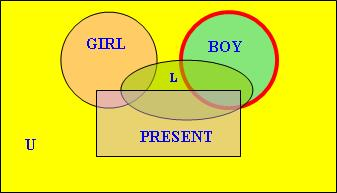(onlyU|BOY)[{x: some(GIRL)({y: LOVE(x, y)})}][PRESENT]     (14)

(QU|S)(A)(B) ⇔ QS(S ∩ A)(S ∩ B)

 ~{x: some(GIRL)({y: LOVE(x, y)})} = BOY ∩ ~{x: some(GIRL)({y: LOVE(x, y)})}} = BOY ∩ {x: no(GIRL)({y: LOVE(x, y)})}} = {x: no(GIRL)({y: LOVE(x, y)})}}

(noU|BOY)[{x: no(GIRL)({y: LOVE(x, y)})}][PRESENT]

Only boys [who love some girl]F are present. ⇔ No boys [who love no girl]F are present.

### 5.3 左論元否定不動點

Q~l ≡ Q     (15)

Q(non-A)(B) ⇔ Q(A)(B)     (16)

 定理5： 設p、q為分數，其中0 ≤ p ≤ q ≤ 1並且p + q = 1，則「<1,1>型量詞」"(constitute between p and q)"和"(constitute more than p but less than q)"是「左論元否定不動點」。特別地，當p = q = 1/2時，p和q滿足上述條件，因此"(constitute exactly half)"也是「左論元否定不動點」。 定理6： 「左論元否定不動點」在「布爾運算」下是封閉的，即若Q和Q'是「左論元否定不動點」，則~Q、(Q ∧ Q')和(Q ∨ Q')也是「左論元否定不動點」。 定理7： 設Q為「<1,1>型量詞」，則「布爾量詞」(Q ∧ Q~l)和(Q ∨ Q~l)是「左論元否定不動點」。

Only or no books [which have been read by at least half of the boys]F will be bought.
⇔ Only or no books [which have been read by less than half of the boys]F will be bought.

### 5.4 左右論元否定

Q~lr(A)(B) ≡ Q(~A)(~B)

Q(A)(B) ⇔ Q~lr(non-A)(not B)     (17)

every
only
(all except John)
(apart from John only)

(onlyU|PARTICIPANT)[{x: some(MISTAKE)({y: MAKE(x, y)})}][{z: no(PRIZE)({w: RECEIVE(z, w)})}]

Q~lr ≡ Q     (18)

Q(non-A)(not B) ⇔ Q(A)(B)     (19)

 定理8： 「左右論元否定不動點」在「布爾運算」下是封閉的，即若Q和Q'是「左右論元否定不動點」，則~Q、(Q ∧ Q')和(Q ∨ Q')也是「左右論元否定不動點」。 定理9： 設Q為「<1,1>型量詞」，則「布爾量詞」(Q ∧ Q~lr)和(Q ∨ Q~lr)是「左右論元否定不動點」。

### 5.5 其他否定概念

「對偶」和「左右論元否定」是兩種否定的複合，前者是「外部否定」與「右論元否定」的複合，後者則是「左論元否定」與「右論元否定」的複合。一個自然的推論是，應該還可定義其他複合否定概念。首先，我們可以把「外部否定」與「左論元否定」複合，從而得到一種新的「對偶」。為區別新舊兩種「對偶」，現把此前介紹的「對偶」改稱「右對偶」(Right Dual)，並把本小節介紹的「對偶」稱為「左對偶」(Left Dual)，記作Qld (而Q的「右對偶」則記作Qrd)，其定義為

Qld(A)(B) ≡ ~(Q(~A)(B))

Q(A)(B) ⇔ It is not the case that Qld(non-A)(B)     (20)

only
some
(constitute more than m of the n)
(constitute at least (n − m) of the n)
(constitute fewer than m of the n)
(constitute at most (n − m) of the n)
(constitute exactly (n − m) of the n)
(constitute more or fewer than m of the n)
(constitute all except m of the n)
(constitute more or fewer than m of the n)
(constitute between l and m of the n)
(constitute more than (n − l) or fewer than (n − m) of the n)
(constitute more than l but fewer than m of the n)
(constitute at least (n − l) or at most (n − m) of the n)
(constitute more than q)
(constitute at least (1 − q))
(constitute less than q)
(constitute at most (1 − q))
(constitute exactly (1 − q))
(constitute more or less than q)
(constitute all except q)
(constitute more or less than q)
(constitute between p and q)
(constitute more than (1 − p) or less than (1 − q))
(constitute more than p but less than q)
(constitute at least (1 − p) or at most (1 − q))

Members constitute at least 2/3 of the participants.
⇔ It is not the case that non-members constitute more than 1/3 of the participants.

Qld ≡ Q     (21)

It is not the case that Q(non-A)(B) ⇔ Q(A)(B)     (22)

Qlrd(A)(B) ≡ ~(Q(~A)(~B))

Q(A)(B) ⇔ It is not the case that Qld(non-A)(not B)     (23)

every
(not only)
only
(not all)

Qlrd ≡ Q

~Q(#) ≡ Q(#)

~Q(#)(B) ⇔ Q(#)(B)

## 6 對當立方體

(every non-)(A)(B) ⇔ ~A ⊆ B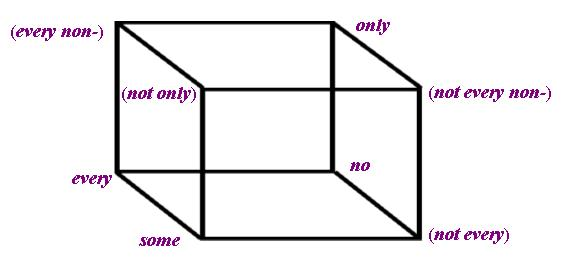IDRNONRDLNLRNLDLRD
IDIDRNONRDLNLRNLDLRD
RNRNIDRDONLRNLNLRDLD
ONONRDIDRNLDLRDLNLRN
RDRDONRNIDLRDLDLRNLN
LNLNLRNLDLRDIDRNONRD
LRNLRNLNLRDLDRNIDRDON
LDLDLRDLNLRNONRDIDRN
LRDLRDLDLRNLNRDONRNID

Cube(Q) = {Q, Q~r, ~Q, Qrd, Q~l, Q~lr, Qld, Qlrd}

Cube(every) = {every, no, (not every), some, (every non-), only, (not every non-), (not only)}

## 7. 對偶三段論

 前提1： Q(A)(B) (24) (註10) 前提2： Q(A)d(C) 結論： some(A)(B ∩ C)

 前提1： 超過三分二會員出席了會議。 前提2： 至少三分一會員是女性。 結論： 有會員是女性且出席了會議。

Q(A)(B) ⇔ Q(A)(A ∩ B)

Q是「左守恆」(Left Conservative)的當且僅當

Q(A)(B) ⇔ Q(A ∩ B)(B)

 前提1： Q(A)(B) (25) 前提2： Qld(C)(B) 結論： some(A ∩ C)(B)

 前提1： 只有會員出席了會議。 前提2： 有女性出席了會議。 結論： 有女會員出席了會議。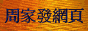<script type = "text/javascript"> (function(d, w) { var x = d.getElementsByTagName('SCRIPT'); var f = function() { var _id = 'lexity-pixel'; var _s = d.createElement('script'); _s.id = _id; _s.type = 'text/javascript'; _s.async = true; _s.src = "//np.lexity.com/embed/YW/be0aa169de7f441c6473361be62c9ef6?id=ddad453e7753"; if (!document.getElementById(_id)) { x.parentNode.insertBefore(_s, x); } }; w.attachEvent ? w.attachEvent('onload', f) : w.addEventListener('load', f, false); }(document, window)); </script>